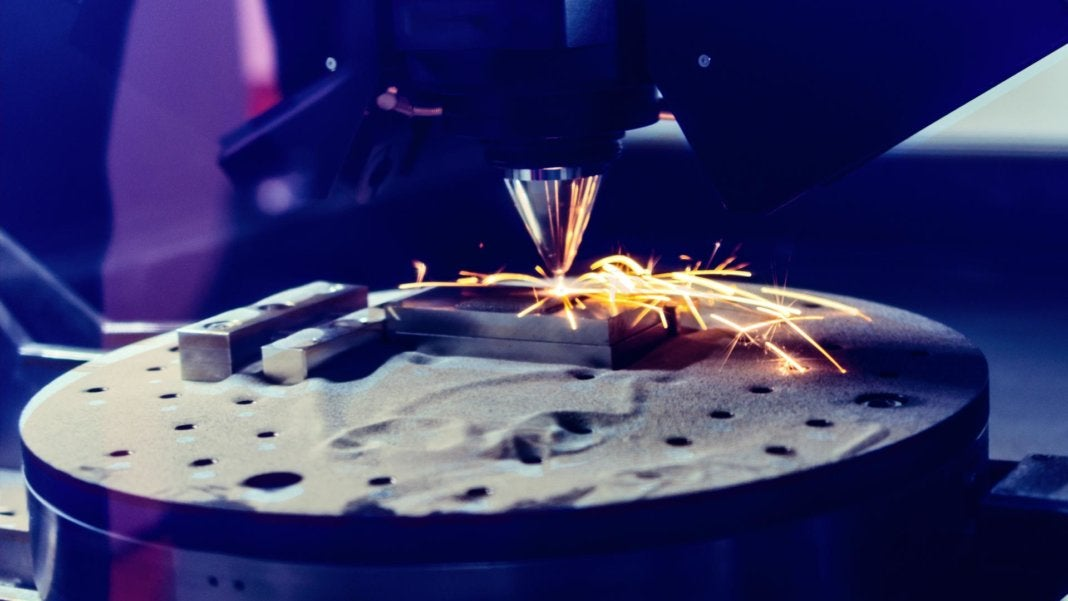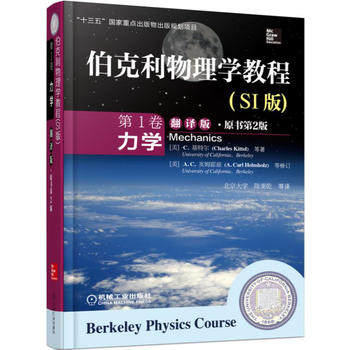我们有望见证3D打印的5大突破3D打印又称增材制造，是这样一种制造成型新工艺，计算机根据数字文件指令，将一层层薄薄的原材料叠起来，最终得到一个空间实物。3D打印机的喷头可以精确地向指定方向释放物质，制造出复杂的结构，从珠宝到三层楼的住宅，都可以打印出来。3D打印珠宝- 阅读剩余部分 -

质点碰撞损失多少动能？《伯克利物理学教程·力学》翻译版封面

《伯克利物理学教程·力学》第6章习题10：

- 阅读剩余部分 -

狗知不知道牛顿运动定律？《夸克与美洲豹》封面

摩擦对于卫星运动的影响《伯克利物理学教程·力学》翻译版封面

《伯克利物理学教程·力学》第6章习题2：

- 阅读剩余部分 -

盖尔曼的科学启蒙- 阅读剩余部分 -

质心参考系中处理两个粒子的弹性碰撞《伯克利物理学教程·力学》翻译版封面\begin{equation} M_1v_1=M_1v'_1\cos\theta_1+M_2v'_2\cos\theta_2 \label{momentumconsx} \end{equation}

\begin{equation} 0=M_1v'_1\sin\theta_1+M_2v'_2\sin\theta_2 \label{momentumconsy} \end{equation}

\begin{equation} \frac{1}{2}M_1v_1^2=\frac{1}{2}M_1{v'}_1^2 + \frac{1}{2}M_2{v'}_2^2 \label{energycons} \end{equation}

- 阅读剩余部分 -

水与水分子波的动图推导惯性系之间的坐标变换- 阅读剩余部分 -

由伽利略不变性推导动量守恒定律- 阅读剩余部分 -